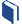# Which Model Models Best?

###Resources for this lesson:

> Glossary> Calculator Resources> Teacher Resources: Instructional NotesWhy might a trigonometric function be the best fit for the data?

The tide data represents a data set that will repeat values over a period of 12 hours.  Since this scenario contains periodic data, a trigonometric (sinusoidal) function should be the best choice for a function to model the data.

## Test and Confirm

• Graph the sinusoidal curve of best fit on the scatter plot.
• Calculate and review the residual plot to determine if the sinusoidal model is the best fit.

When ready, move to the next page.